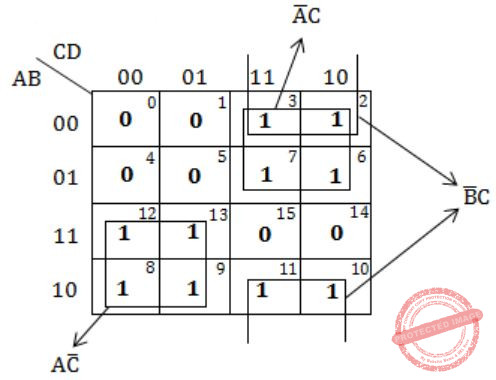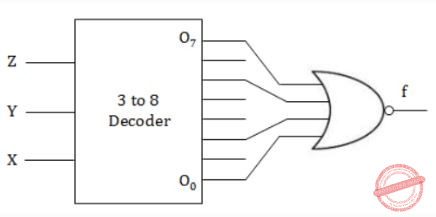## GATE 2008-IT

 Question 1

A set of Boolean connectives is functionally complete if all Boolean functions can be synthesized using those. Which of the following sets of connectives is NOT functionally complete?

 A EX-NOR B implication, negation C OR, negation D NAND
Digital-Logic-Design       Boolean-Functions
Question 1 Explanation:
→ EX-NOR is not functionally complete.
→ NOR and NAND are the functionally complete logic gates, OR, AND, NOT only logic gate can be implemented by using them.
→ And (Implication, Negation) is also functionally complete.
 Question 2

A sample space has two events A and B such that probabilities P(A ∩ B) = 1/2, P(A') = 1/3, P(B') = 1/3. What is P(A ∪ B)?

 A 11/12 B 10/12 C 9/12 D 8/12
Engineering-Mathematics       Probability
Question 2 Explanation:
P(A ∩ B) = 1/2
P(A') = 1/3; P(A) = 2/3
P(B') = 1/3; P(B) = 2/3
P(A ∪ B) = P(A) +P(B) - P(A ∩ B)
= 2/3 + 2/3 - 1/2
= 4+4-3/ 6
= 5/6
= 10/12
 Question 3

What is the chromatic number of the following graph?A 2 B 3 C 4 D 5
Engineering-Mathematics       Graph-Theory
Question 3 Explanation:
Chromatic number = 3
→ Chromatic number of a graph is the smallest number of colours needed to colour the vertices so that no two adjacent vertices share the same colour.Question 4

What is the size of the smallest MIS(Maximal Independent Set) of a chain of nine nodes?

 A 5 B 4 C 3 D 2
Engineering-Mathematics       Graph-Theory
Question 4 Explanation:
1 - 2 - 3 - 4 - 5 - 6 - 7 - 8 - 9
(2, 5, 8) is the maximal independent set for a chain of 9 nodes. If we add any start node to the set then it will not be MIS.
Independent set:
A set of vertices is called independent set such that no two vertices in the set are adjacent.
 Question 5

Which of the following regular expressions describes the language over {0, 1} consisting of strings that contain exactly two 1’s?

 A (0 + 1) * 11(0 + 1) * B 0 * 110 * C 0 * 10 * 10 * D (0 + 1) * 1(0 + 1) * 1 (0 + 1) *
Theory-of-Computation        Regular-Expressions
Question 5 Explanation:
Option A: (0+1)* 11(0+1)*
→ 1110 this consists of 3 ones but accepted by given expression. So this is false.
Option B: 0* 110*
→ 0101 this string consists of two is but not accepted by given expression. This is false.
Option C: 0* 10* 10*
→ This is true.
Option D: (0+1)* 1(0+1)* 1(0+1)*
→ 011010 - This can have three is but accepted by given expression. So this is also false.
 Question 6

Let N be an NFA with n states and let M be the minimized DFA with m states recognizing the same language. Which of the following in NECESSARILY true?

 A m ≤ 2n B n ≤ m C M has one accept state D m = 2n
Theory-of-Computation       Finite-Automata
Question 6 Explanation:
Set of states of NFA = n
A state in a DFA is a proper subset of states of NFA of corresponding DFA.
→ No. of subsets with n elements = 2n
→ m ≤ 2n
 Question 7

The following bit pattern represents a floating point number in IEEE 754 single precision format

` 110000011101000000000000000000000`
The value of the number in decimal form is
 A -10 B -13 C -26 D None of these
Digital-Logic-Design       Number-Systems
Question 7 Explanation:
Sign bit is 1 then given number is negative.
Exponent bits - 10000011
Exponent can be added with 127 bias in IEEE single precision format then outval exponent
= 10000011 - 127
= 131 - 127
= 4
→ In IEEE format, an implied 1 is before mantissa, and hence the outval number is
→ 1.101 × 24 = -(11010)2 = -26
 Question 8

Consider the following Boolean function of four variables

` f(A,B,C,D) = Σ(2, 3, 6, 7, 8, 9, 10, 11, 12, 13)`
The function is
 A independent of one variable B independent of two variables C independent of three variable D dependent on all the variables
Digital-Logic-Design       Boolean-Functions
Question 8 Explanation:
f(A, B, C, D) = Σ(2, 3, 6, 7, 8, 9, 10, 11, 12, 13)Independent of one variable '0'.
 Question 9

What Boolean function does the circuit below realize ?A xz+x’z’ B xz’+x’z C x’y’+yz D xy+y’z’
Digital-Logic-Design       Decoder
Question 9 Explanation:
f = (x’y’z’ + x’yz’+xy’z+xyz)’
= (x’z’ + xz)’
= x’z + xz’
 Question 10

Arrange the following functions in increasing asymptotic order:

```A. n1/3
B. en
C. n7/4
D. n log9n
E. 1.0000001n```
 A A, D, C, E, B B D, A, C, E, B C A, C, D, E, B D A, C, D, B, E
Algorithms        Time-Complexity
Question 10 Explanation:
A < D < C < E < B
n1/3 < n log9 < n7/4 < 1.0000001n < en
 Question 11

For problems X and Y, Y is NP-complete and X reduces to Y in polynomial time. Which of the following is TRUE?

 A If X can be solved in polynomial time, then so can Y B X is NP-complete C X is NP-hard D X is in NP, but not necessarily NP-complete
Algorithms        P-NP
Question 11 Explanation:
Note: Out of syllabus.
 Question 12

Which of the following is TRUE?

 A The cost of searching an AVL tree is θ (log n) but that of a binary search tree is O(n) B The cost of searching an AVL tree is θ (log n) but that of a complete binary tree is θ (n log n) C The cost of searching a binary search tree is O (log n) but that of an AVL tree is θ(n) D The cost of searching an AVL tree is θ (n log n) but that of a binary search tree is O(n)
Data-Structures       AVL-Trees
Question 12 Explanation:
Complexity of AVL tree is O(logn) because it is a balanced tree, in Worst case binary search tree complexity is O(n).
 Question 13

Match the programming paradigms and languages given in the following table.A I-c, II-d, III-b, IV-a B I-a, II-d, III-c, IV-b C I-d, II-c, III-b, IV-a D I-c, II-d, III-a, IV-b
Programming       Match-the-Following
Question 13 Explanation:
Note: Out of syllabus.
 Question 14

Consider the execution of the following commands in a shell on a linux operating system.

```system
bash\$cat alpha
Mathematics
bash\$in alpha beta
bash\$ rm alpha
bash\$cat >> beta << SAME
Information Technology
SANE
bash\$ cat beta```
The output of the last command will be:
 A Mathematics Information Technology SAME. B Mathematics Information Technology. C Information Technology. D Information Technology SAME.
Operating-Systems       LINUX
Question 14 Explanation:
Note: Out of syllabus.
 Question 15

A processor that has carry, overflow and sign flag bits as part of its program status word (PSW) performs addition of the following two 2's complement numbers 01001101 and 11101001. After the execution of this addition operation, the status of the carry, overflow and sign flags, respectively will be:

 A 1, 1, 0 B 1, 0, 0 C 0, 1, 0 D 1, 0, 1
Digital-Logic-Design       Number-Systems
Question 15 Explanation:Carry flag = 1
Overflow flag = 0
Sign bit = 0 (MSB bit is 0)
Overflow flag:
In computer processors, the overflow flag is usually a single bit in a system status register used to indicate when an arithmetic overflow has occurred in an operation.
 Question 16

A paging scheme uses a Translation Look-aside Buffer (TLB). A TLB-access takes 10 ns and a main memory access takes 50 ns. What is the effective access time(in ns) if the TLB hit ratio is 90% and there is no page-fault?

 A 54 B 60 C 65 D 75
Operating-Systems        Virtual Memory
Question 16 Explanation:
EMAT = TLB access time + Miss ratio × Main memory access time + Main memory access time
= 10 + 0.1 × 50 + 50
= 10 + 5 + 50
= 65
 Question 17

Find if the following statements in the context of software testing are TRUE or FALSE.
(S1) Statement coverage cannot guarantee execution of loops in a program under test.
(S2) Use of independent path testing criterion guarantees execution of each loop in a program under test more than once.

 A True, True B True, False C False, True D False, False
Question 17 Explanation:
Note: Out of syllabus.
 Question 18

How many bytes of data can be sent in 15 seconds over a serial link with baud rate of 9600 in asynchronous mode with odd parity and two stop bits in the frame?

 A 10,000 bytes B 12,000 bytes C 15,000 bytes D 27,000 bytes
Computer-Organization       Serial-Communication
Question 18 Explanation:
In the Asynchronous mode of transmission along with per byte, we need to send some extra bit like start, stop bit and parity bit, etc.
1 bit for start bit, 8 bits for data, 1 bit for parity, 2 bits for stop bits i.e.,Question 19

Which of the following is TRUE only of XML but NOT HTML?

 A It is derived from SGML B It describes content and layout C It allows user defined tags D It is restricted only to be used with web browsers
Web-Technologies       XML
Question 19 Explanation:
Note: Out of syllabus.
 Question 20

Provider the best matching between the entries in the two columns given in the table below:A I-a, II-d, III-c, IV-b B I-b, II-d, III-c, IV-a C I-a, II-c, III-d, IV-b D I-b, II-c, III-d, IV-a
Computer-Networks       Match-the-Following
Question 20 Explanation:
(i) Proxy server: Proxy server and firewall are to be combined.
(ii) Kazaa and DC++ are P2P applications.
(iii) P2P slip is a processor of PPP.
(iv) DNS allows caching of entries at local server.
 Question 21

Which of the following first order formula is logically valid? Here α(x) is a first order formula with x as a free variable, and β is a first order formula with no free variable.

 A [β→(∃x,α(x))]→[∀x,β→α(x)] B [∃x,β→α(x)]→[β→(∀x,α(x))] C [(∃x,α(x))→β]→[∀x,α(x)→β] D [(∀x,α(x))→β]→[∀x,α(x)→β]
Engineering-Mathematics       Propositional-Logic
Question 21 Explanation:
[(∃x,α(x))→β]→[∀x,α(x)→β]
L.H.S. : If there is an x such that α(x) is true, then β is true.
R.H.S. : For all x, if α(x) true, then β is true.
Here, the given LHS and RHS are to be same as β is a formula which can be independent of x (if β is true for one x, it is true for every x, and vice-versa).
Here, LHS = RHS
So, Option C is valid.
There are 21 questions to complete.

Register Now